# Rhombus

PQRS is a rhombus. Given that PQ=3 cm & height of rhombus is given 2 cm. Calculate its area.

Result

S =  6 cm2

#### Solution:

$S = ah = 3 \cdot 2 = 6 \ cm^2$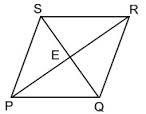Our examples were largely sent or created by pupils and students themselves. Therefore, we would be pleased if you could send us any errors you found, spelling mistakes, or rephasing the example. Thank you!

Leave us a comment of this math problem and its solution (i.e. if it is still somewhat unclear...):Be the first to comment!## Next similar math problems:

1. Acreage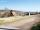Plot has a diamond shape, its side is 25.6 m long and the distance of the opposite sides is 22.2 meters. Calculate its acreage.
2. Rhombus 4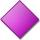Circumference of the rhombus is 44 cm, its height is 89 mm long. Calculate its content area.
3. Unknown numberIdentify unknown number which 1/5 is 40 greater than one tenth of that number.
4. Pipe cross sectionThe pipe has an outside diameter 1100 mm and the pipe wall is 100 mm thick. Calculate the cross section of this pipe.
5. CableCable consists of 8 strands, each strand consists of 12 wires with diameter d = 0.5 mm. Calculate the cross-section of the cable.
6. TVsProduction of television sets increased from 3,500 units to 4,200 units. Calculate the percentage of production increase.
7. GardenThe garden around the new majer is divided as follows: 35% vegetable, 30% fruit orchards, 10% flowers and the remaining 120 m2 are lawns. What is the total area of the garden?
8. Tiles path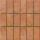A rectangular park measures 24mX18m. A path 2.4m wide is constructed all around it. Find the cost of paving the path with cement tiles of size 60cm X 40cm at the rate of \$5.60 per tile ?
9. Megapascals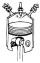What is the area of crosssection of the piston, if the force of 300 kN produces a pressure of 5 MPa?
10. Two gardenersThe garden with an area of 81 square meters was divided by two gardeners in a ratio of 4: 5. How much did the second gardener get more first?
11. Trapezium 2Trapezium has an area of 24 square cms. How many different trapeziums can be formed ?
12. ClassIn a class are 32 pupils. Of these are 8 boys. What percentage of girls are in the class?
13. EquationSolve the equation: 1/2-2/8 = 1/10; Write the result as a decimal number.
14. InequationSolve the inequation: 5k - (7k - 1)≤ 2/5 . (5-k)-2
15. Percents - easyHow many percent is 432 out of 434?
16. Conference148 is the total number of employees. The conference was attended by 22 employees. How much is it in percent?
17. Base, percents, valueBase is 344084 which is 100 %. How many percent is 384177?